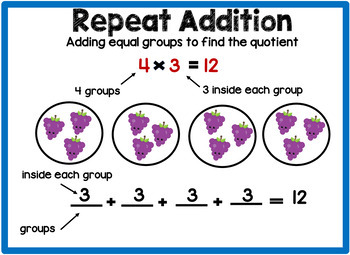# Multiplication and Division Anchor Chart Posters Bundle in English & SpanishSubject
Resource Type
File Type

Zip

(21 MB|90 pages)
Product Rating
Standards
2 Products in this Bundle
2 products
1. These multiplication anchor charts in English and Spanish are displayed in my classroom as a bulletin board for my English Language Learners. When I teach a multiplication strategy I use one of these anchor charts to introduce the strategy then display it so that my bilingual students can reference
2. These division anchor charts in English and Spanish are displayed in my classroom as a bulletin board for my English Language Learners. When I teach a division strategy, I use one of these anchor charts to introduce the strategy then display it so that my bilingual students can reference it while s
Also included in:
1. These Math anchor charts in English and Spanish are displayed in my classroom as a bulletin board for my English Language Learners. When I teach one of the basic operations strategies I use one of these anchor charts to introduce the strategy. I then display it so that my bilingual students can re
\$20.00
\$16.00
Save \$4.00
• Bundle Description
• StandardsNEW

These multiplication and division anchor charts in English and Spanish are displayed in my classroom as a bulletin board for my English Language Learners. When I teach a multiplication strategy or a division strategy I use one of these anchor charts to introduce the strategy. I then display it so that my bilingual students can reference it while solving a word problem. Included are multiplication tables from 1 - 12 for students to copy for morning work or reference when they are trying to solve a problem. These anchor charts can also be used in an English as a Second Language classroom or a Dual Language classroom.

The multiplication anchor charts are part of the Third Grade Math Anchor Chart Posters Bundle in my store.

Fluently multiply and divide within 100, using strategies such as the relationship between multiplication and division (e.g., knowing that 8 × 5 = 40, one knows 40 ÷ 5 = 8) or properties of operations. By the end of Grade 3, know from memory all products of two one-digit numbers.
Apply properties of operations as strategies to multiply and divide. Examples: If 6 × 4 = 24 is known, then 4 × 6 = 24 is also known. (Commutative property of multiplication.) 3 × 5 × 2 can be found by 3 × 5 = 15, then 15 × 2 = 30, or by 5 × 2 = 10, then 3 × 10 = 30. (Associative property of multiplication.) Knowing that 8 × 5 = 40 and 8 × 2 = 16, one can find 8 × 7 as 8 × (5 + 2) = (8 × 5) + (8 × 2) = 40 + 16 = 56. (Distributive property.)
Use multiplication and division within 100 to solve word problems in situations involving equal groups, arrays, and measurement quantities, e.g., by using drawings and equations with a symbol for the unknown number to represent the problem.
Interpret whole-number quotients of whole numbers, e.g., interpret 56 ÷ 8 as the number of objects in each share when 56 objects are partitioned equally into 8 shares, or as a number of shares when 56 objects are partitioned into equal shares of 8 objects each. For example, describe a context in which a number of shares or a number of groups can be expressed as 56 ÷ 8.
Interpret products of whole numbers, e.g., interpret 5 × 7 as the total number of objects in 5 groups of 7 objects each. For example, describe a context in which a total number of objects can be expressed as 5 × 7.
Total Pages
90 pages
N/A
Teaching Duration
N/A
Report this Resource to TpT
Reported resources will be reviewed by our team. Report this resource to let us know if this resource violates TpT’s content guidelines.